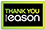local_shipping Spend over €10 for free home delivery  place Free 2 Hour Click & Collect Service

# Handbook of differential equations

Hardback
Category: Mathematics
Handbook of Differential Equations: Evolutionary Equations is the last text of a five-volume reference in mathematics and methodology. This volume follows the format set by the preceding volumes, presenting numerous contributions that reflect the nature of the area of evolutionary partial differential equations. The book is comprised of five chapters that feature the following: A thorough discussion of the shallow-equations theory, which is used as a model for water waves in rivers, lakes and oceans. It covers the issues of modeling, analysis and applications. Evaluation of the singular limits of reaction-diffusion systems, where the reaction is fast compared to the other processes; and applications that range from the theory of the evolution of certain biological processes to the phenomena of Turing and cross-diffusion instability Detailed discussion of numerous problems arising from nonlinear optics, at the high-frequency and high-intensity regime Geometric and diffractive optics, including wave interactions Presentation of the issues of existence, blow-up and asymptotic stability of solutions, from the equations of solutions to the equations of linear and non-linear thermoelasticity Answers to questions about unique space, such as continuation and backward uniqueness for linear second-order parabolic equations. Research mathematicians, mathematics lecturers and instructors, and academic students will find this book invaluable.
€259.00777 Reward Points
Currently out of stock
Delivery 5-7 Days

Any purchases for more than €10 are eligible for free delivery anywhere in the UK or Ireland!

Handbook of Differential Equations: Evolutionary Equations is the last text of a five-volume reference in mathematics and methodology. This volume follows the format set by the preceding volumes, presenting numerous contributions that reflect the nature of the area of evolutionary partial differential equations. The book is comprised of five chapters that feature the following: A thorough discussion of the shallow-equations theory, which is used as a model for water waves in rivers, lakes and oceans. It covers the issues of modeling, analysis and applications. Evaluation of the singular limits of reaction-diffusion systems, where the reaction is fast compared to the other processes; and applications that range from the theory of the evolution of certain biological processes to the phenomena of Turing and cross-diffusion instability Detailed discussion of numerous problems arising from nonlinear optics, at the high-frequency and high-intensity regime Geometric and diffractive optics, including wave interactions Presentation of the issues of existence, blow-up and asymptotic stability of solutions, from the equations of solutions to the equations of linear and non-linear thermoelasticity Answers to questions about unique space, such as continuation and backward uniqueness for linear second-order parabolic equations. Research mathematicians, mathematics lecturers and instructors, and academic students will find this book invaluable.
Currently out of stock
Delivery 5-7 Days777 Reward Points

Any purchases for more than €10 are eligible for free delivery anywhere in the UK or Ireland!

€259.00
Currently out of stock
Delivery 5-7 Days777 Reward Points

Any purchases for more than €10 are eligible for free delivery anywhere in the UK or Ireland!

### Product Description

Handbook of Differential Equations: Evolutionary Equations is the last text of a five-volume reference in mathematics and methodology. This volume follows the format set by the preceding volumes, presenting numerous contributions that reflect the nature of the area of evolutionary partial differential equations. The book is comprised of five chapters that feature the following: A thorough discussion of the shallow-equations theory, which is used as a model for water waves in rivers, lakes and oceans. It covers the issues of modeling, analysis and applications. Evaluation of the singular limits of reaction-diffusion systems, where the reaction is fast compared to the other processes; and applications that range from the theory of the evolution of certain biological processes to the phenomena of Turing and cross-diffusion instability Detailed discussion of numerous problems arising from nonlinear optics, at the high-frequency and high-intensity regime Geometric and diffractive optics, including wave interactions Presentation of the issues of existence, blow-up and asymptotic stability of solutions, from the equations of solutions to the equations of linear and non-linear thermoelasticity Answers to questions about unique space, such as continuation and backward uniqueness for linear second-order parabolic equations. Research mathematicians, mathematics lecturers and instructors, and academic students will find this book invaluable.

### Product Details

#### Handbook of differential equations

ISBN9780444532220

FormatHardback

Publisher (29 May. 2009)

No. of Pages534

Weight1140

Language English (United States)

Dimensions 241 x 175